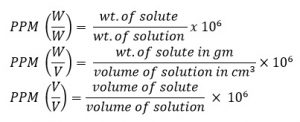# Concentration Of Solution

Concentration of solution corresponds to the amount of solute present in the given amount of solution. There are different units to explain the concentration of solution.

Units of concentration:
1. Percentage of solution:
It is the no. of part of solute present in 100 parts of solution. Percentage of solution can be expressed either in W/W, W/V or V/V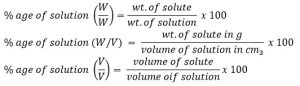2. Normality of Solution (eqv.l-1 or N)
It is defined as the no. of gram eqv. of solute present in 1L of its solution.
Mathematically,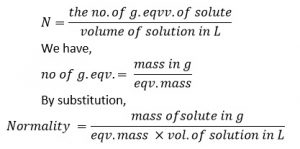A solution is said to have concentration 1 normal (1N), decinormal (N/10) and centinormal (N/100) as 1,0.1,0.01 gm eqv. Of solute are present in 1L of solution respectively.

3. Molarity of solution (moml L-1 or M)
It is defined as the no. of g. mol. Of solute present in 1L of its solution.
Mathematically,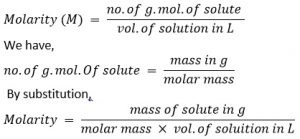A solution is said to have concentration 1 molar (1M), decimolar (M/10) and centimolar (M/100) as 1, 0.1 and 0.01 g. mol. Of solute are present in 1L of its solution respectively.

4. Gram/L (gL-1)
It is defined as the no. of gm of solute present in 1L of solution. gL-1 can be related to normality (N) and molarity (M) ad thereby normality and molarity can be related to one another and it is reduced as follows: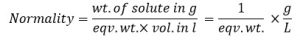gL-1 = Normality x eqv. wt. → 1
Similarly,
gL-1 = molarity x mol. Wt. → 2

then from equation 1 and 2. We get,
Normality x eqv. wt. = molarity x mol. Wt.

This expression says, normality and molarity of solution becomes identical only if eq. wt. and mol. wt. are same.

5. Molarity (mol Kg-1 or m)
Molarity is defined as the no. of moles of solute present in 1 kg. of solvent.
Mathematically,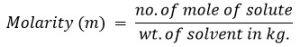A solution is said to have 1 molar concentration when 1 mole of solute is present in 1 kg of solvent.

6. Mole Fraction (x)
Mole fraction is the fraction of total no. of mole of a compound present in the mixture.
Let us consider a solution containing n1 mole of solvent and n2 mole of solute.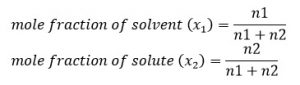Here, x1 + x2 = 1

7. Part per million (PPM)
Part per million is the no. of part of solute present in 1 million part of solution.